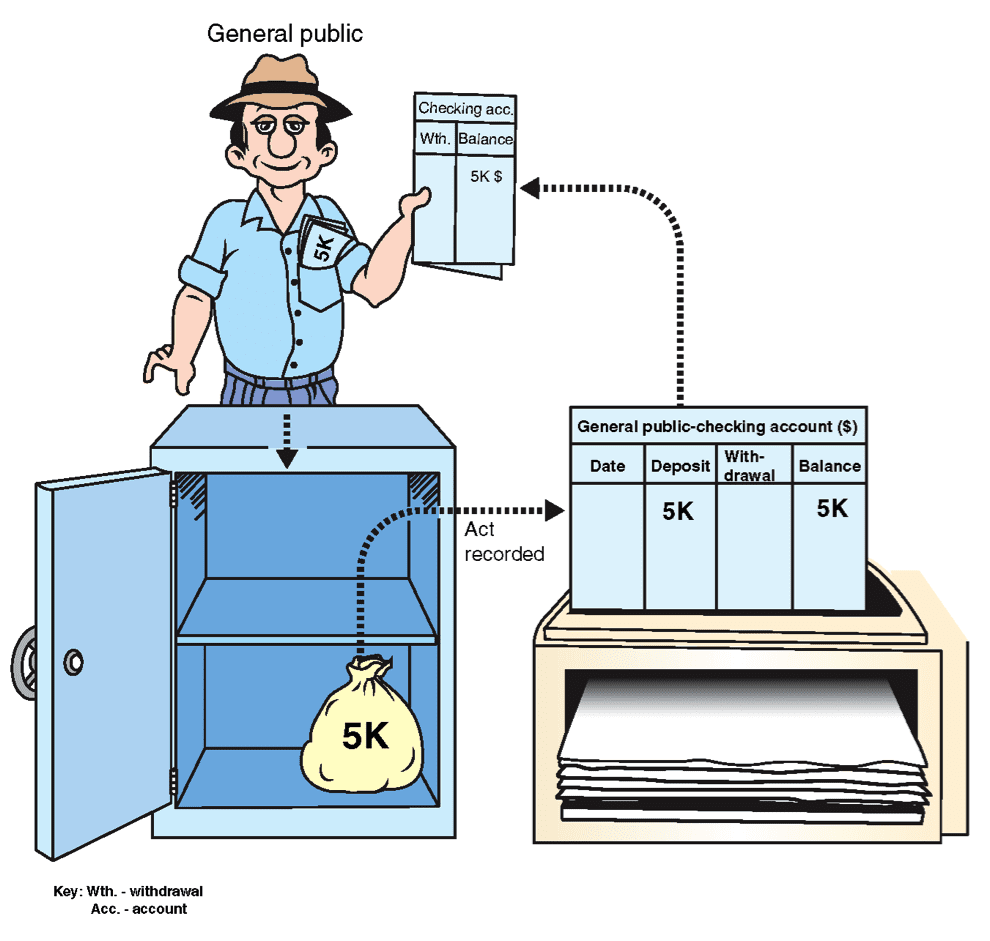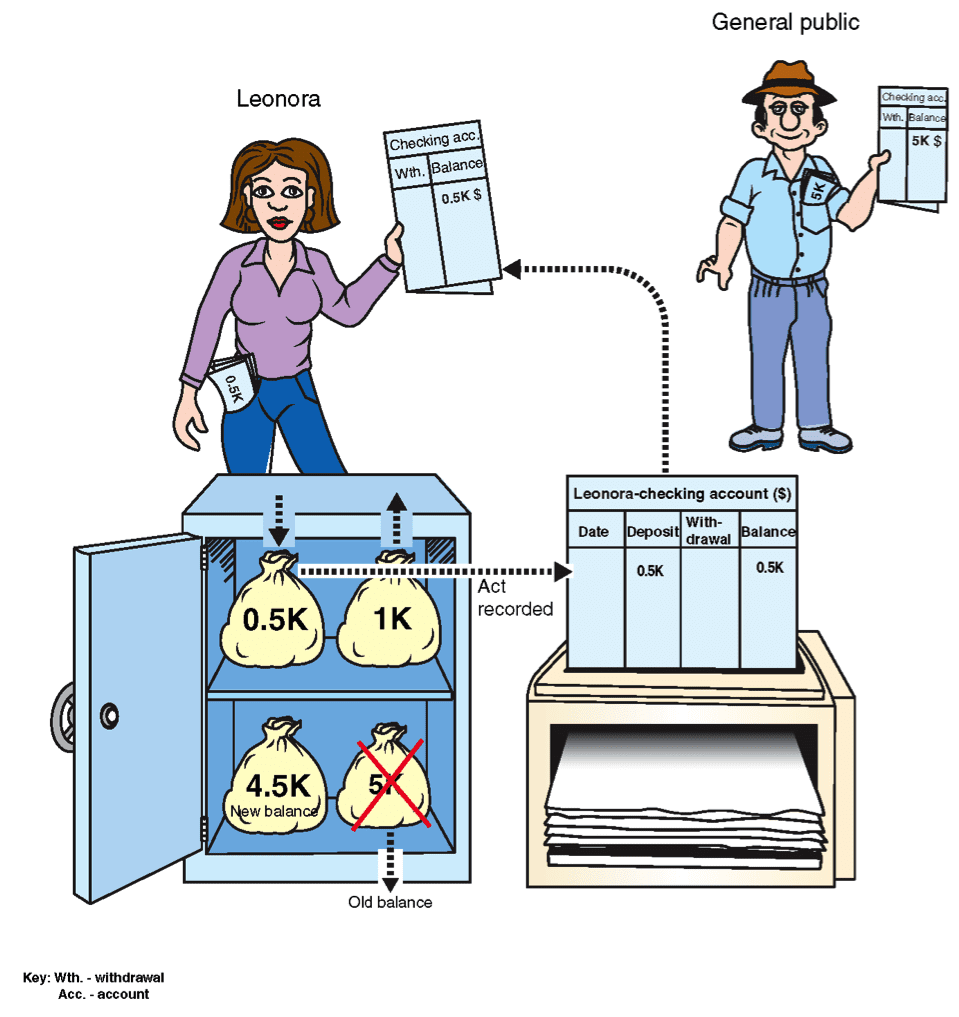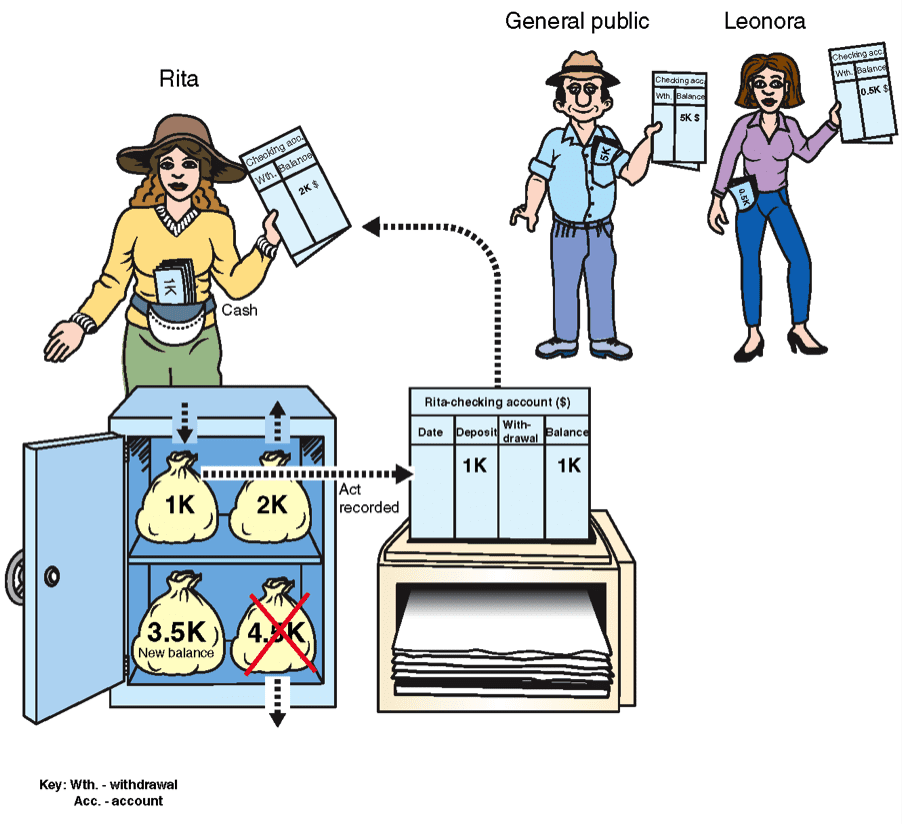# The Reserve Ratio

## The Maximum Amount of Loans

Can the bank continue to make loans endlessly? The answer is no – the amount is limited.

The bank can make loans as long as it does not fall below a threshold measured by a ratio called the “reserve ratio”, the meaning of which will be explained shortly. The reserve ratio is set by the central bank in each country.

## The Reserve Ratio-Definition

 Reserve Ratio = Total Cash in the Bank Total Checking Account Balance

Example:

In the bank in Country A, after making the third loan to Golden Hotel Company (Illustration 7.10), the reserve ratio is 0.5, according to the following calculation:

 0.5    = \$10,000 (Total Cash in the Bank) \$20,000 (= 7k+2k+1k+10k = Total Checking Account Balance)

In other words, for each dollar on deposit in a checking account, the bank holds a reserve of 0.5 dollars. The rest is available for lending.

## Setting the Reserve Ratio

In every country there is a body that is called the central bank (explained below), which sets the minimum reserve ratio that the bank must preserve. The minimum reserve ratio represents the amount that the bank must hold in its vault for every \$1 in its checking accounts. The bank can, however, keep more in its safe if it wishes.

If the central bank decides that the bank must keep a minimum reserve ratio of 0.5, then the bank must have \$0.50 in its vault for every \$1 on deposit in its checking accounts. If the checking account balances in the bank total \$1 million, the bank must keep at least \$0.5 million in its vault.

If the reserve ratio in Country A (above) is set at 0.25 then the bank can continue to make loans until the total balance in its checking accounts reaches \$40,000. Only then will the bank reach the 0.25 reserve ratio limit.

 0.25    = Total Cash in the Bank : \$10,000 Total Checking Account Balance : \$40,000

Making a Bank Loan When the Country is in Situation II-

Situation II – 50% of the inhabitants’ money is held in cash at all times, and 50% in checking accounts.

In Situation II, the inhabitants of the country keep 50% of their money in cash and 50% in checking accounts. Assume that the reserve ratio is 0.25.  The starting point is shown in Illustration 7.11.

Illustration 7.11First Loan

Leonora receives a \$1,000 loan. Since the country is in Situation II, she keeps 50% in cash and deposits 50% in a checking account. The up-to-date situation is shown in Illustration 7.12.

Illustration 7.12Explanation

Leonora receives \$1,000 in cash (the cash is removed from the bank’s vault). She deposits \$500 in a checking account (this amount is returned to the bank’s vault), and keeps the rest. The total money supply now increases to \$11,000: \$5.5k in checking account balances and \$5.5k in cash (the cash in the illustration is protruding from her pocket).

The reserve ratio is 0.82 = (Total Cash in the Bank: \$4,500)/ (Total Checking Account Balance\$5,500)

Second Loan

Rita receives a \$2,000 loan. Since the country is in Situation II, she keeps \$1,000 in cash, and deposits \$1,000 in a checking account.

The situation at this stage is shown in Illustration 7.13.

Illustration 7.13The money supply has grown to \$13,000.

The reserve ratio is 0.54 =(Total Cash in the Bank \$3,500)/ (Total Checking Account Balance \$6,500)

In other words, the bank has \$0.54 in its vault for every \$1 in a checking account.